# Class 11 Maths NCERT Solutions for Chapter 10 Straight Lines Exercise 10.2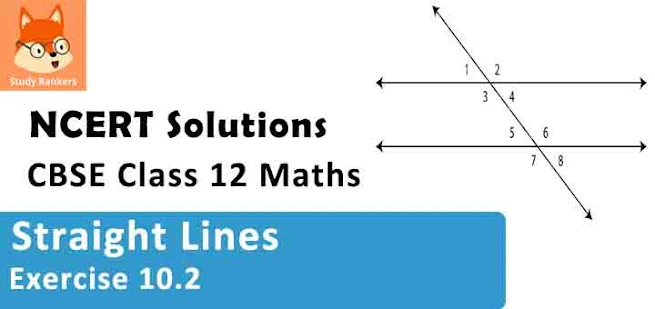### Straight Lines Exercise 10.2 Solutions

1. Consider the given population and year graph. Find the slope of the line AB and using it, find what will be the population in the year 2010?

Solution

The y-coordinate of every point on the x-axis is 0.
Therefore, the equation of the x-axis is y = 0.
The x-coordinate of every point on the y-axis is 0.
Therefore, the equation of the y-axis is x = 0.

2. Find the equation of the line which passes through the point (–4, 3) with slope 1/2 .

Solution

We know that the equation of the line passing through point (x0 ,y0), whose slope is m, is (y - y0) = m(x - x0).
Thus, the equation of the line passing through point (-4, 3), whose slope is 1/2, is
(y - 3) = 1/2 (x + 4)
⇒ 2(y - 3) = x + 4
⇒ 2y - 6 = x + 4
i.e., x - 2y + 10 = 0

3. Find the equation of the line which passes though (0, 0) with slope m.

Solution

We know that the equation of the line passing through point(x0 , y0), whose slope is m, is (y - y0) = m(x - x0).
Thus, the equation of the line passing through point (0, 0), whose slope is m, is
(y - 0) = m(x - 0)
i.e., y = mx

4. Find the equation of the line which passes though (2, 2√3) and is inclined with the x-axis at an angle of 75° .

Solution

The slope of the line that inclines with the x-axis at an angle of 75° is
m = tan 75°We know that the equation of the line passing through point (x0 , y0), whose slope  is m, is (y - y0) = m(x - x0).
Thus, if a line passes though (2, 2√3) and inclines with the x - axis at an angle of 75° , then the equation of the line is given as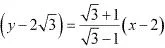⇒ (y - 2√3)(√3  1) = (√3 + 1)(x - 2)
⇒ y(√3 - 1) - 2√3(√3 - 1) = x(√3 + 1) - 2(√3 + 1)
⇒ (√3 + 1)x - (√3 - 1)y = 2√3 + 2 - 6 + 2√3
⇒ (√3 + 1)x - (√3 - 1)y = 4√3 - 4
i.e., (√3 + 1)x - (√3 - 1)y = 4(√3 - 1)

5. Find the equation of the line which intersects the x-axis at a distance of 3 units to the left of origin with slope –2.

Solution

It is known that if a line with slope m makes x-intercept d, then the equation of the line is given as
y = m(x – d)
For the line intersecting the x-axis at a distance of 3 units to the left of the origin, d = –3.
The slope of the line is given as m = –2
Thus, the required equation of the given line is
y = –2 [x – (–3)]
⇒ y = –2x – 6
i.e., 2x + y + 6 = 0

6. Find the equation of the line which intersects the y-axis at a distance of 2 units above the origin and makes an angle of 30° with the positive direction of the x-axis.

Solution

It is known that if a line with slope m makes y-intercept c, then the equation of the line is given as
y = mx + c
Here, c = 2 and m = tan 30°. 1/√3
Thus, the required equation of the given line is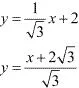⇒ √3y = x + 2√3
i.e., x - √3y + 2√3 = 0

7. Find the equation of the line which passes through the points (–1, 1) and (2, –4).

Solution

It is known that the equation of the line passing through points (x1, y1) and (x2, y2) is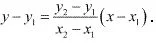Therefore, the equation of the line passing through the points (-1, 1) and (2, -4) is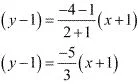⇒ 3(y - 1) = -5(x+ 1)
⇒ 3y - 3 = -5x - 5
i.e., 5x + 3y + 2 = 0

8. Find the equation of the line which is at a perpendicular distance of 5 units from the origin and the angle made by the perpendicular with the positive x-axis is 30°

Solution

If p is the length of the normal from the origin to a line and ω is the angle made by the normal with the positive direction of the x-axis, then the equation of the line is given by xcos ω + y sin ω = p.
Here, p = 5 units and ω = 30°
Thus, the required equation of the given line is
x cos 30° + y sin 30° = 5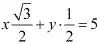i.e., √3x + y = 10

9. The vertices of ΔPQR are P (2, 1), Q (–2, 3) and R (4, 5). Find equation of the median through the vertex R.

Solution

It is given that the vertices of ΔPQR are P (2, 1), Q (–2, 3), and R (4, 5).
Let RL be the median through vertex R.
Accordingly, L is the mid-point of PQ.
By mid-point formula, the coordinates of point L are given by [(2 -2)/2, (1 + 3)/2] = (0, 2)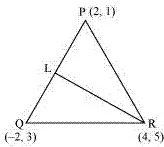It is known that the equation of the line passing through points (x1 ,y1) and (x2 , y2) isTherefore, the equation of RL can be determined by substituting (x1 ,y1) = (4, 5)
and (x2 ,y2) = (0, 2).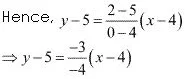⇒ 4(y - 5) = 3(x - 4)
⇒ 4y - 20 = 3x - 12
⇒ 3x - 4y + 8 = 0
Thus, the required equation of the median through vertex R is 3x - 4y + 8 = 0.

10. Find the equation of the line passing through (–3, 5) and perpendicular to the line through the points (2, 5) and (–3, 6).

Solution

The slope of the line joining the points (2, 5) and (–3, 6) is m = (6 -5)/(-3 -2) = 1/-5
We know that two non-vertical lines are perpendicular to each other if and only if their slopes are negative reciprocals of each other.
Therefore, slope of the line perpendicular to the line through the points (2, 5) and (–3, 6) = -1/m = 1/(-1/5) = 5
Now, the equation of the line passing through point (–3, 5), whose slope is 5, is
(y - 5) = 5(x + 3)
y - 5 = 5x + 15
i.e., 5x - y + 20 = 0

11. A line perpendicular to the line segment joining the points (1, 0) and (2, 3) divides it in the ratio 1:n. Find the equation of the line.

Solution

According to the section formula, the coordinates of the point that divides the line segment joining the points (1, 0) and (2, 3) in the ratio 1: n is given by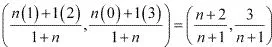The slope of the line joining the points, (1, 0) and (2, 3) is
m = (3 - 0)/(2 - 1) = 3
We know that two non - vertical lines are perpendicular to each other if and only if their slopes are negative reciprocals of each other.
Therefore, slope of the line that is perpendicular to the line joining the points (1, 0)
and (2, 3) = -1/m = -1/3
Now, the equation of the line passing through [(n + 2)/(n + 1) , 3/(n + 1)] and whose slope  is -1/3 is given by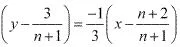⇒  3[(n + 1) y - 3] = -[x(n + 1) - (n + 2)]
⇒ 3(n + 1)y - 9 = -(n + 1)x + n + 2
⇒ (1 + n)x + 3(1 + n)y = n + 11

12. Find the equation of a line that cuts off equal intercepts on the coordinate axes and passes through the point (2, 3).

Solution

The equation of a line in the intercept form is
x/a + y/b = 1 ...(i)
Here, a and b are the intercepts on x and y axes respectively.
It is given that the line cuts off equal intercepts on both the axes. This means that a = b.
Accordingly, equation (i) reduces to
x/a + y/b = 1
⇒ x + y = a ...(ii)
Since the given line passes through point (2, 3), equation (ii) reduces to
2 + 3 = a ⇒ a = 5
On substituting the value of a in equation (ii), we obtain
x + y = 5, which is the required equation of the line.

13. Find equation of the line passing through the point (2, 2) and cutting off intercepts on the axes whose sum is 9.

Solution

The equation of a line in the intercept form is
x/a + y/b = 1 ...(i)
Here, a and b are the intercepts on x and y axes respectively.
It is given that a + b = 9 ⇒ b = 9 - a  ...(ii)
From equations (i) and (ii), we obtain
x/a + y/(9 - a) = 1  ...(iii)
It is given that the line passes through point (2, 2). Therefore, equation (iii) reduces to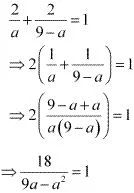⇒18 = 9a – a2
⇒ a2 - 9a + 18 = 0
⇒ a2 - 6a - 3a + 18  = 0
⇒ a(a - 6) - 3 (a - 6) = 0
⇒ (a - 6)(a - 3) = 0
⇒ a = 6 or a = 3
If a = 6 and b = 9 - 6 = 3, then the equation of the line is
x/6 + y/3 = 1 ⇒ x + 2y - 6 = 0
If a = 3 and b = 9 - 3 = 6, then the equation of the line is
x/3 + y/6 = 1 ⇒ 2x + y - 6 = 0

14. Find equation of the line through the point (0, 2) making an angle 2π/3 with the positive x-axis. Also, find the equation of line parallel to it and crossing the y-axis at a distance of 2 units below the origin.

Solution

The slope of the line making an angle 2π/3 with the positive x - axis is m = tan (2π/3) = -√3
Now, the equation of the line passing through point (0, 2) and having a slope -√3 is (y - 2) = -√3(x - 0)
y - 2 = -√3x
i.e., √3x + y - 2 = 0
The slope of line parallel to line √3x + y - 2 = 0 is -√3.
It is given that the line parallel to line √3x + y - 2 = 0 crosses the y - axis 2 units below the origin i.e., it passes through point (0, -2).
Hence, the equation of the line passing through point (0, -2) and having a slope -√3 is
y -(-2) = -√3(x - 0)
⇒ y + 2 = -√3x
⇒ √3x + y + 2 = 0

15. The perpendicular from the origin to a line meets it at the point (– 2, 9), find the equation of the line.

Solution

The slope of the line joining the origin (0, 0) and point (-2, 9) is m1 = (9 - 0)/(-2 -0) = -9/2
Accordingly, the slope of the line perpendicular to the line joining the origin and point (-2, 9) is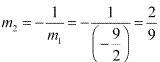Now, the equation of the line passing through point (-2, 9) and having a slope m2 is
(y - 9) = 2/9 (x + 2)
⇒ 9y - 81 = 2x + 4
i.e., 2x - 9y + 85 = 0

16. The length L (in centimetre) of a copper rod is a linear function of its Celsius temperature C. In an experiment, if L = 124.942 when C = 20 and L = 125.134 when C = 110, express L in terms of C

Solution

It is given that when C = 20, the value of L is 124.942, whereas when C = 110, the value of L is 125.134.
Accordingly, points (20, 124.942) and (110, 125.134) satisfy the linear relation between L and C.
Now, assuming C along the x-axis and L along the y-axis, we have two points i.e., (20, 124.942) and (110, 125.134) in the XY plane.
Therefore, the linear relation between L and C is the equation of the line passing through points (20, 124.942) and (110, 125.134).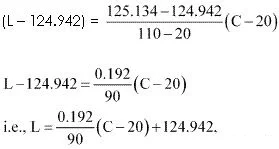which is the required linear relation.

17. The owner of a milk store finds that, he can sell 980 litres of milk each week at Rs 14/litre and 1220 litres of milk each week at Rs 16/litre. Assuming a linear relationship between selling price and demand, how many litres could he sell weekly at Rs 17/litre?

Solution

The relationship between selling price and demand is linear.
Assuming selling price per litre along the x-axis and demand along the y-axis, we have two points i.e., (14, 980) and (16, 1220) in the XY plane that satisfy the linear relationship between selling price and demand.
Therefore, the linear relationship between selling price per litre and demand is the equation of the line passing through points (14, 980) and (16, 1220).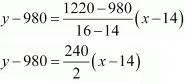⇒ y - 980 = 120(x - 14)
i.e., y = 120(x - 14) + 980
When x = Rs 17/litre,
y = 120(17 - 14) + 980
⇒ y = 120 × 3 + 980 360 + 980 = 1340
Thus, the owner of the milk store could sell  1340 litres milk weekly at Rs  17/litre.

18. P (a, b) is the mid-point of a line segment between axes. Show that equation of the line is x/a + y/b = 2

Solution

Let AB be the line segment between the axes and let P(a, b) be its mid - point.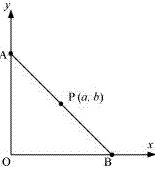Let the coordinates of A and B be (0, y) and (x, 0) respectively.
Since P (a, b) is the mid - point of AB,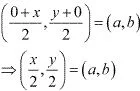⇒ x/2 = a and  y/2 = b
∴ x = 2a and y = 2b
Thus, the respective coordinates of A and B are (0, 2b) and (2a, 0).
The equation of the line passing through points (0, 2b) and (2a, 0) is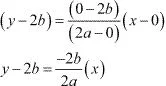⇒ a(y - 2b) = -bx
⇒ ay - 2ab = -bx
i.e., bx + ay = 2ab
On dividing both sides by ab, we obtain
bx/ab + ay/ab = 2ab/ab
⇒ x/a + y/b = 2
Thus, the equation of the line is x/a + y/b = 2.

19. Point R (h, k) divides a line segment between the axes in the ratio 1:2. Find equation of the line.

Solution

Let AB be the line segment between the axes such that point R (hk) divides AB in the ratio 1: 2.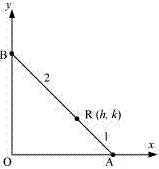Let the respective coordinates of A and B be (x, 0) and (0, y).
Since point R (h, k) divides AB in the ratio 1 : 2, according to the section formula,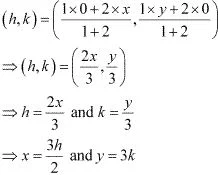Therefore, the respective coordinates of A and B are (3h/2, 0) and (0, 3k).
Now, the equation of line AB passing through points (3h/2, 0) and (0, 3k) is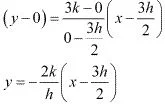hy = -2kx + 3hk
i.e., 2kx  + hy = 3hk
Thus, the required equation of the line is 2ky + hy = 3hk.

20. By using the concept of equation of a line, prove that the three points (3, 0), (–2, –2) and (8, 2) are collinear.

Solution

In order to show that points (3, 0), (–2, –2), and (8, 2) are collinear, it suffices to show that the line passing through points (3, 0) and (–2, –2) also passes through point (8, 2).
The equation of the line passing through points (3, 0) and (–2, –2) is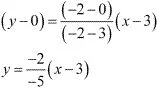5y = 2x - 6
i.e., 2x - 5y = 6
It is observed that at x = 8 and y = 2,
L.H.S. = 2×8 - 5×2 = 16 - 10 = 6 = R.H.S.
Therefore, the line passing through points (3, 0) and (-2, -2) also passes through point (8, 2). Hence, points (3, 0). (-2, -2) and (8, 2) are collinear.